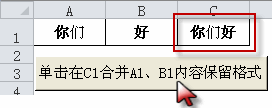# 解决公式不能实现excel保留格式的方法

[日期：2011-12-15]   来源：IT部落窝  作者：IT部落窝   阅读：1015[字体： ]

这是群里的一个问题：单元格连接内容的同时如何做到excel保留格式。

具体的excel保留格式效果如下：A1单元格里面的“你”是加粗的，“们”未加粗；B1单元格里面的“好”为加粗。excel保留格式的最终效果为C1单元格的效果。

实现这个效果，在excel工作表按下ALT+F11，打开VBE编辑器，插入――模块，复制下面的代码，将代码指定给按钮即可。

Sub excel保留格式()
Dim x As Range, n%, i%, m
[c1] = [a1] & [b1]
For Each x In [a1].Resize(1, 3)
If x.Column = 1 Then m = 0 Else m = m + Len(x.Offset(0, -1))
For i = 1 To Len(x)
n = m + i
Debug.Print n
[c1].Characters(n, 1).Font.ColorIndex = x.Characters(i, 1).Font.ColorIndex
[c1].Characters(n, 1).Font.Bold = x.Characters(i, 1).Font.Bold
Next
Next
End Sub分享到： QQ空间 新浪微博 腾讯微博 人人网